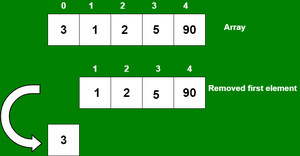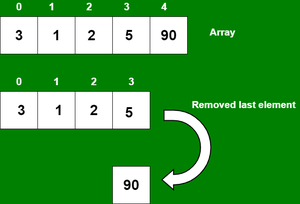Open In App

# How to delete an element from an array using JavaScript ?

The array is a type of data structure that allows us to store similar types of data under a single variable. The array is helpful to create a list of elements of similar types, which can be accessed using their index.

In this article, we will discuss different ways to remove elements from the array. There are many methods that are used to remove elements from the JavaScript array which are discussed below.

We can delete an element or item from an array using two functions which is:

• JavaScript Array shift() Method
• JavaScript Array pop() Method
• Using JavaScript splice() Method
• Using JavaScript Array filter() Method

## Remove Array elements by using Array shift() Method

This method is used to delete one or more elements from the array and these elements get deleted from the beginning of the array because of the deleted elements or items from the array, the length of the array also gets decreased by the number of elements deleted from the array.

Below are examples of how to do this: Let’s see how to delete an element at the beginning of the array using the shift() function.

```Input:  arr[] = {3, 1, 2, 5, 90}, size = 5, capacity = 5
Output: arr[] = {_, 1, 2, 5, 90}, size = 4, capacity = 5```

Example 1: In this example, we use an array to delete elements at the beginning of an array using the shift() function.## Javascript

 `const arr = [3, 1, 2, 5, 90];``arr.shift();``console.log(arr);`

Output

```[ 1, 2, 5, 90 ]
```

Example 2: In this example, we use an associative array to delete elements at the beginning of an array using the JavaScript array shift() function.

## Javascript

 `let arr = [``    ``{ key: ``'first'``, val: ``'3'` `},``    ``{ key: ``'second'``, val: ``'1'` `},``    ``{ key: ``'third'``, val: ``'2'` `},``    ``{ key: ``'fourth'``, val: ``'5'` `},``    ``{ key: ``'fifth'``, val: ``'90'` `}``];``arr.shift();``console.log(arr);`

Output

```[
{ key: 'second', val: '1' },
{ key: 'third', val: '2' },
{ key: 'fourth', val: '5' },
{ key: 'fifth', val: '90' }
]
```

## Remove elements by using the JavaScript array pop() Method

This method is used to pop or delete elements or items from an array. We can pop one or more elements from the array and these elements get deleted from the end of the array because of the popped elements from the array, the length of the array also gets decreased by the number of elements popped from the array.

Below are examples of how to do it: Let’s see how to delete an element at the end of the array using the pop() function.

```Input:  arr[] = {3, 1, 2, 5, 90}, size = 5, capacity = 5
Output: arr[] = {3, 1, 2, 5, _}, size = 4, capacity = 5```

Example 1: In this example, we use an array to delete elements at the end of an array using the JavaScript array pop() function.## Javascript

 `let arr = [3, 1, 2, 5, 90];` `console.log(``"Orginal array: "` `+ arr);``console.log(``"Extracted element: "` `+ arr.pop());``console.log(``"Remaining elements: "` `+ arr);`

Output

```Orginal array: 3,1,2,5,90
Extracted element: 90
Remaining elements: 3,1,2,5
```

Example 2: In this example, we use an associative array to delete elements at the end of an array using the pop() function.

## Javascript

 `let arr = [``    ``{ key: ``'first'``, val: 3 },``    ``{ key: ``'second'``, val: 1 },``    ``{ key: ``'third'``, val: 2 },``    ``{ key: ``'fourth'``, val: 5 },``    ``{ key: ``'fifth'``, val: 90 }``];` `let val = arr.pop();` `console.log(``'key popped: '` `+ val.key);``console.log(``'value popped: '` `+ val.val);`

Output

```key popped: fifth
value popped: 90
```

## Delete an Element from Array using JavaScript splice() Method

The JavaScript Array splice() Method is an inbuilt method in JavaScript that is used to modify the contents of an array by removing the existing elements and/or by adding new elements.

Example:

## Javascript

 `function` `func() {``    ``let array = [``"HTML"``, ``"CSS"``, ``"JS"``, ``"Bootstrap"``];`` ` `    ``console.log(``"Original array: "` `+ array);``                 ` `    ``// Add 'React' and 'Php' after removing 'JS'.``    ``let removedElement = array.splice(1, 1, ``'PHP'``, ``'React'``)``                 ` `    ``console.log(``"Array after splice: "` `+ array);``    ``console.log(``"Removed element: "` `+ removedElement);                           ``}``func();`

Output

```Original array: HTML,CSS,JS,Bootstrap
Array after splice: HTML,PHP,React,JS,Bootstrap
Removed element: CSS
```

## Delete an Array Element using JavaScript Array filter() Method

The JavaScript Array filter() Method is used to create a new array from a given array consisting of only those elements from the given array which satisfy a condition set by the argument method.

Example:

## Javascript

 `// Declare and initialize an array``let array = [``"algo"``, ``"maths"``, ``"science"``, ``"biology"``]``             ` `// Using filter method to create a remove method``function` `arrayRemove(arr, value) {``    ``return` `arr.filter(``function` `(geeks) {``        ``return` `geeks != value;``    ``});            ``}`` ` `let result = arrayRemove(array, ``"algo"``);``console.log(``"Remaining elements: "` `+ result)`

Output

```Remaining elements: maths,science,biology
```